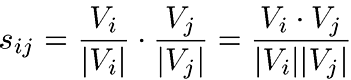Next:Harris's Tree Structure AnalysisUp:Constructing a Domain Model Previous:The Syntagmatic Approach

## The Paradigmatic Approach

The purpose of paradigmatic analysis is to find the most similar terms in the corpus. The similarity of every two terms is calculated by comparing the frequencies of terms next to them on their left and right sides. Similarities are scaled between -1 and 1, where 1 means identical occurences and -1 completely different occurences.

Each term i is in the analysis represented by a vector $V$i, the elements of which have been obtained from a vector containing frequences of terms next to i, through applying to each of its elements the formula (1) used in the syntagmatic analysis. The vector is then divided with its own length, so that the length of the resulting vector $V$i becomes 1. The similarity index of two such vectors $V$i and$V$j is the dot product of the two vectors:Calculation of this formula requires optimization, which will not be described in this paper.

Terms with high similarity (represented by the arbitrary symbols $i$ and $j$) are distributed very similarly and their syntactic properties resemble each other very much. In a grammar, this could be presented by rules which produce these terms from the same arbitrary nonterminal symbol (e.g. $U$->i and $U$-> j).Next:Harris's Tree Structure AnalysisUp:Constructing a Domain Model Previous:The Syntagmatic Approach
Päivikki Parpola

Sat Oct 14 22:52:14 EEST 2000